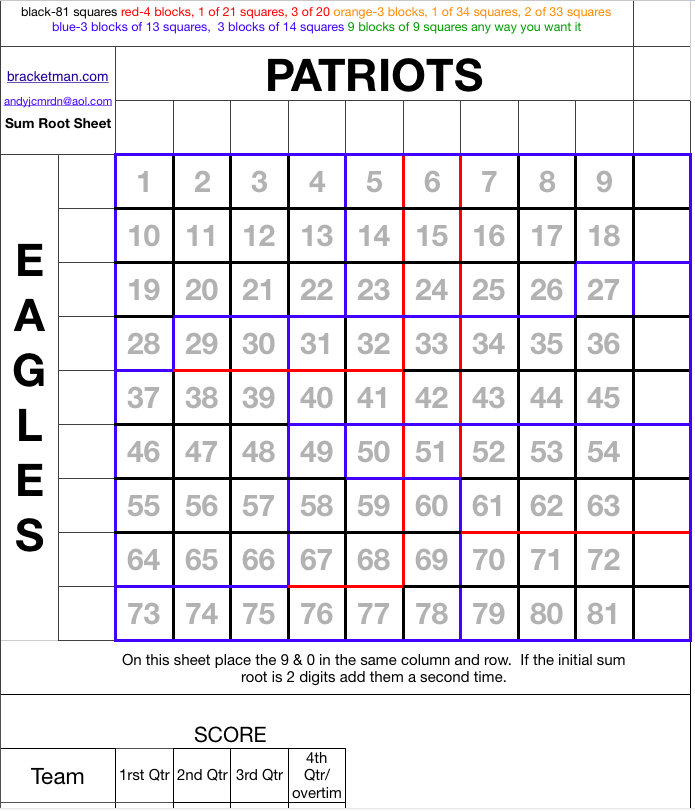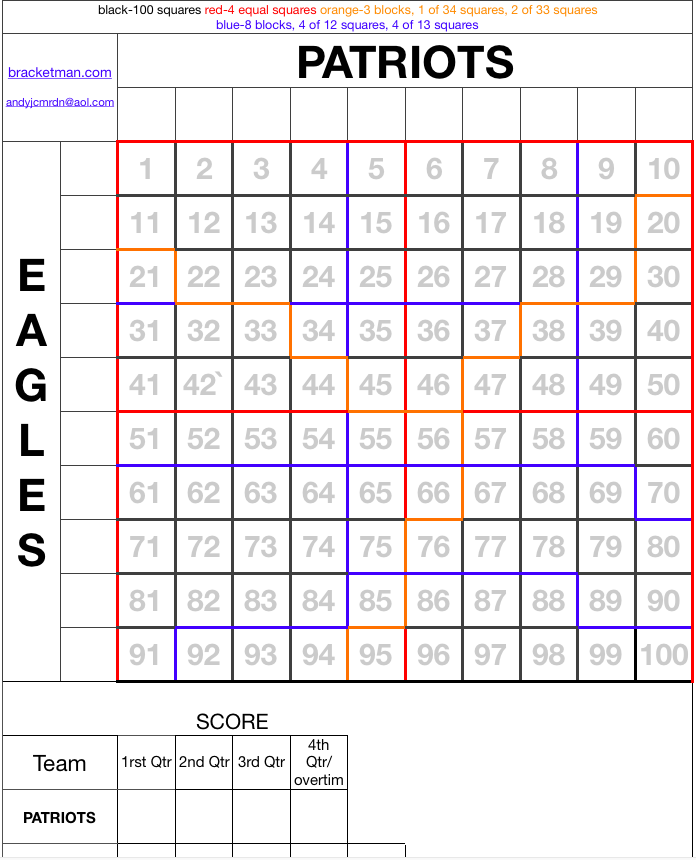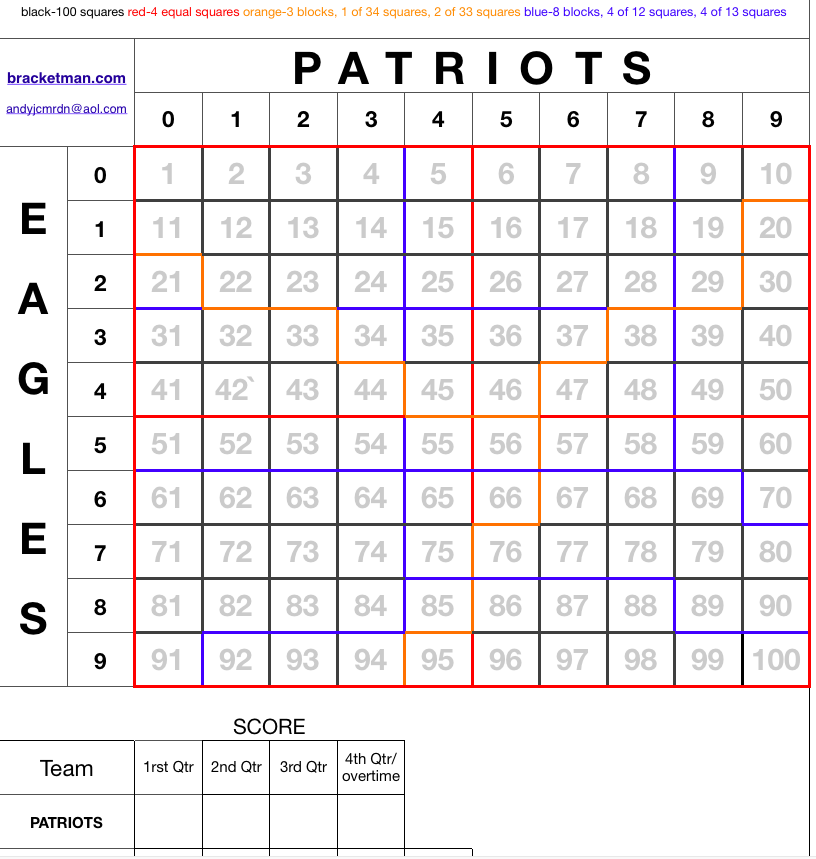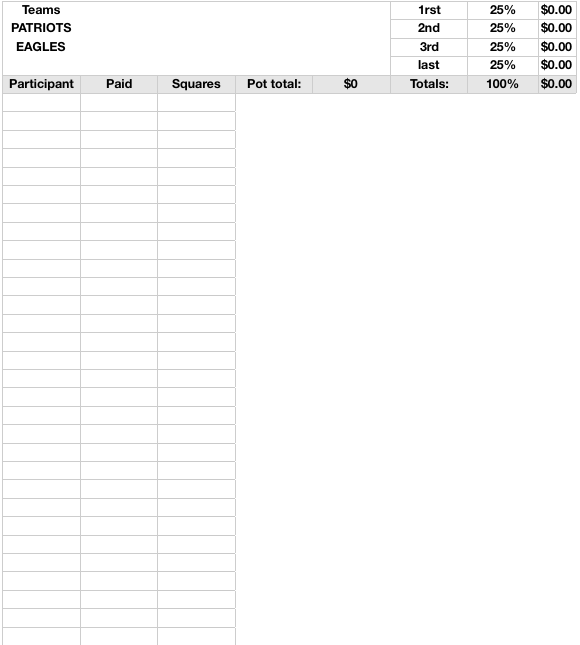15Jan/13

## NFL Super Bowl Squares Sheet

There are three different super bowl square sheets included in this download: Traditional squares sheet with the numbers entered on the axis, Traditional sheet without the numbers entered on the axis and a sum root square sheet for the most equal chances of winning per square.

Instructions: Assign each team to an axis. Sell the squares or the blocks of squares to the participants. Once all the squares are sold then randomnly assign the numbers 0 through 9 to the x-axis and the y-axis. After each quarter of the super bowl take the last digit of each teams score and find the intersecting square on the sheet and the owner of that square wins the money set aside for that quarter.

The background numbers in the squares are to help participants comunicate to you the squares they want to purchase.

Alternatively for a more equal chance for every square to win add the numbers together of each teams score using these numbers to find the intersecting square at the end of each quarter. If the sum of a teams score is two digits add those digits to get a singe digit. Combine the 9 and 0 columns/rows together leaving the sheet with 81 squares as opposed to the traditional 100 squares. See the table below (taken from mathgoespop.com) for the distribution of the likliness of particular number to hit in a superbowl squares game.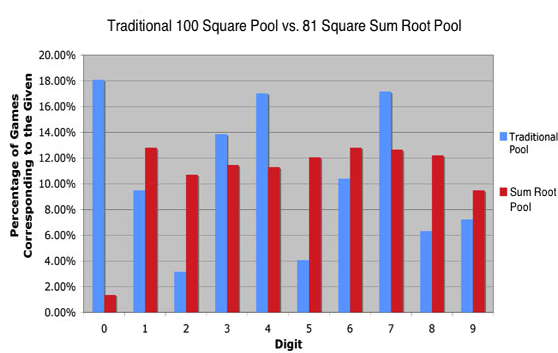SUM ROOT SUPER BOWL SQUARES SHEET